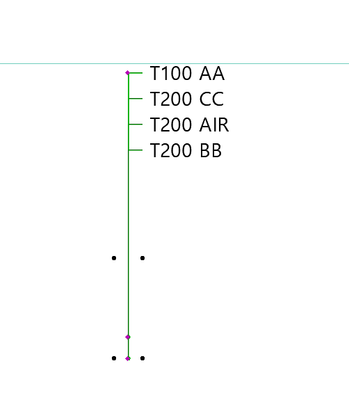cancel
Showing results for
Did you mean:
cancel
Showing results for
Did you mean:
Developer forum

## Can I skip the value from the label to mark it?Contributor

Hi

I'm making a label for Finishing materials.

there is a problem to solve that I want to skip the value named AIR but i don't know how to skip it.For example, in this picture Even if the slab contains 4 materials, I don't want to label the material named AIR.

this is the scripts i used.

 for i = 1 to numTextif (fra(MATthk[n]*1000) > -eps and fra(MATthk[n]*1000) < eps) thenthk = str("%.0mm", MATthk[n])elsethk = str("%.1mm", MATthk[n])endif autotxt[i] = "T" + thk + " " + MATname[n]autoTHK[i] = MATthk[n]

I don't see how to exclude the value MATname[n] = "AIR" in the middle of this root.

Even if i force it to make it by removing the value, it was only marked as zero, but it was not completely removed.

I want only three expressions: AA, BB, and CC on my label.Like this picture.

thank you.Enthusiast

a simple if statement inside the loop should do the trick:

if (MATname[n] = "Air") then

autotxt[i] =""

else

autotxt[i] = "T" + thk + " " + MATname[n]
autoTHK[i] = MATthk[n]

endif

After that I asume you loop over autotext[] to get the lines of the label, so you can check for autotext[i] lenght and if it is less than 1 you can skip it.Latest solutions

Most liked posts